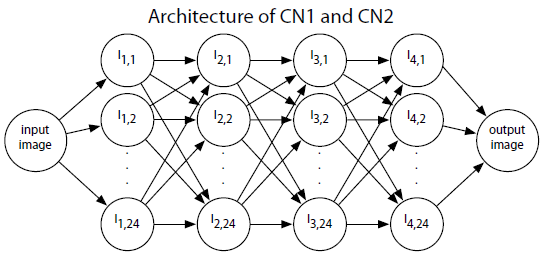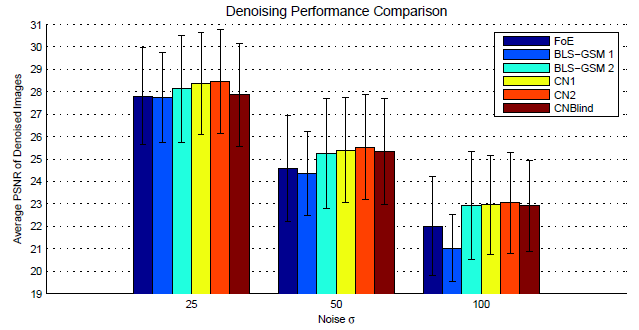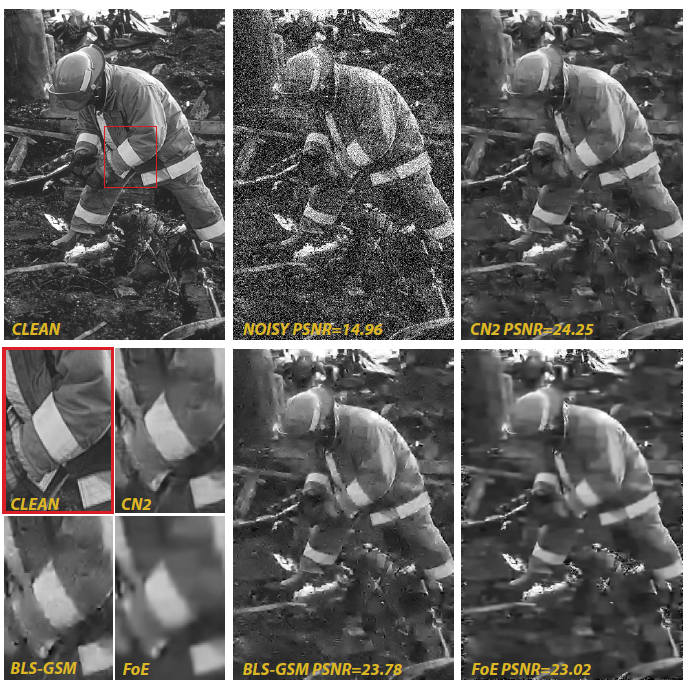# Brief Review — Natural Image Denoising with Convolutional Networks

## Convolutional Network for Image Denoising in Year of 2008

Natural Image Denoising with Convolutional Networks
Jain NIPS’08, by Massachusetts Institute of Technology
2008 NIPS, Over 900 Citations (Sik-Ho Tsang @ Medium)
Image Denoising

# Outline

1. Convolutional Networks for Image Denoising
2. Results

# 1. Convolutional Networks for Image Denoising

## 1.1. Network Architecture

• A convolutional network is an alternating sequence of linear filtering and nonlinear transformation operations. The input and output layers include one or more images, while intermediate layers contain “hidden” units with images called feature maps that are the internal computations of the algorithm.
• The activity of feature map a in layer k is given by:
• with f(x) is sigmoid activation function:
• where Ik-1,b are feature maps that provide input to Ik,a, ⊗ denotes the convolution operation, and θk,a is a bias parameter
• Input and target values are in the range of 0 to 1, and hence the 8-bit integer intensity values of the dataset (values from 0 to 255) were normalized to lie between 0 and 1.
• The border of the image is explicitly encoded by padding an area surrounding the image with values of -1.Architecture of convolutional network used for denoising.
• The network has 4 hidden layers and 24 feature maps in each hidden layer. In layers 2, 3, and 4, each feature map is connected to 8 randomly chosen feature maps in the previous layer.
• Each arrow represents a single convolution associated with a 5×5 filter, and hence this network has 15,697 free parameters and requires 624 convolutions to process its forward pass.

## 1.2. Training Details

• A noise process n(x) is used that operates on an image xi drawn from a distribution of natural images X.
• Reconstruction error is minimized:
• Mini-batch training is used where a gradient update is computed from 6×6 patches randomly sampled from 6 different images in the training set.
• Finally, when learning networks with two or more hidden layers it was important to use a very small learning rate for the final layer (0.001) and a larger learning rate (0.1) in all other layers.

# 2. ResultsDenoising results as measured by peak signal to noise ratio (PSNR) for 3 different noise levels
• CN1 and CNBlind are learned using the same forty image training set as the Field of Experts model (FoE).
• CN2 is learned using a training set with an additional sixty images.

The convolutional network has the highest average PSNR using either training set.Denoising results on an image from the test set (σ=50)
• A visual comparison of these results is shown above.

This paper was appeared after LeNet (1989, 1998), earlier than AlexNet (2012). ReLU is not used. No Caffe, TensorFlow, PyTorch, etc. Awesome!

## Reference

[2008 NIPS] [Jain NIPS’08]
Natural Image Denoising with Convolutional Networks

## Image Restoration

2008 [Jain NIPS’08] 2016 [RED-Net] [GDN] 2017 [DnCNN] [MemNet] [IRCNN] [WDRN / WavResNet] 2018 [MWCNN] 2019 [IDBP-CNN-IA]

--

--